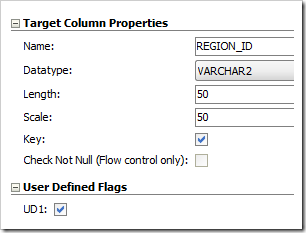# Handling the “ORDER BY” clause in an interface

Hello Everyone…

We always get a lot of emails asking about how to “Order By” in ODI because of that, here there is a simple way to do it…

Stays as a suggestion to Oracle implement as default feature in forward versions!

A simple example how to handle Order By in ODI.

## Solution 1

#### Step 1.  Create an Option#### Step 2. Add the code into KM

```<% if (odiRef.getOption("ORDER_BY").equals("1")) { %>
ORDER BY
<%=odiRef.getColList("", "[COL_NAME]", ",nt", "", "(UD1)")%>
<%=odiRef.getColList(",", "[COL_NAME]", ",nt", "", "(UD2)")%>
<%=odiRef.getColList(",", "[COL_NAME]", ",nt", "", "(UD3)")%>
<%=odiRef.getColList(",", "[COL_NAME]", ",nt", "", "(UD4)")%>
<%=odiRef.getColList(",", "[COL_NAME]", ",nt", "", "(UD5)")%>
<%=odiRef.getColList(",", "[COL_NAME]", ",nt", "", "(UD6)")%>
<%=odiRef.getColList("", "[COL_NAME]", ",nt", "", "(UD7)")%>
<%=odiRef.getColList("", "[COL_NAME]", ",nt", "", "(UD8)")%>
<%=odiRef.getColList("", "[COL_NAME]", ",nt", "", "(UD9)")%>
<%=odiRef.getColList("", "[COL_NAME]", ",nt", "", "(UD10)")%>
<%} %>```

This way you can have the KM to handle with and without Order By option .

#### Step 3.  Mark the Columns accordingly with UD1## Solution 2

#### Step 1.  Create an Option#### Step 2 . Add the option into KM

`<%=odiRef.getOption("ORDER_BY")%>`

#### Step 3.  Add the Order By Clause accordingly

Provide the Order By clause accordingly for N number of columns  in the interfaceSample Output

Good to see you around…

Keep visiting us at www.odiexperts.com